## Example Questions

← Previous 1

### Example Question #1 : How To Divide Exponents

Simplify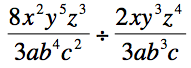Possible Answers: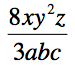None of the answers are correct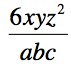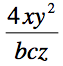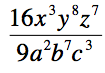Correct answer:Explanation:

When working with polynomials, dividing is the same as multiplying by the reciprocal.  After multiplying, simplify.  The correct answer for division isand the correct answer for multiplication is### Example Question #1 : How To Divide Exponents

Simplify: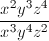Possible Answers: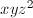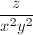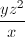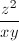Correct answer:Explanation:

To simply exponents in a fraction, subtract the exponent for each variable in the denominator from the exponent in the numerator. This will leave you with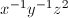or### Example Question #3 : How To Divide Exponents

What is the value of m where: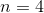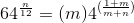Possible Answers:

1

6

-2

4

2

Correct answer:

2

Explanation:

If n=4, then 64(4/12)=64(1/3)=4.  Then, 4=m4(1+m)/(m+4). If 2 is substituted for m, then 4=24(1+2)/(2+4)=241/2=2√4=22=4.

### Example Question #4 : How To Divide Exponents

Simplify the following: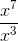Possible Answers:

x7/3

1/x4

Cannot be done

x4

Correct answer:

x4

Explanation:

These exponents have the same base, x, so they can be divided. To divide them, you take the exponent value in the numerator (the top exponent) and subtract the exponent value of the denominator (the bottom exponent). Here that means we take 7 – 3 so our answer is x4.

### Example Question #5 : How To Divide Exponents

Simplify: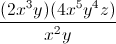Possible Answers: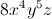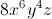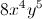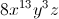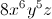Correct answer:Explanation: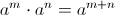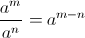Use rule for multiplying exponents to simplify the numerator.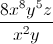Use rule for dividing exponents to simplify.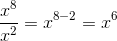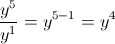### Example Question #6 : How To Divide Exponents

Simplify: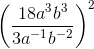Possible Answers: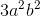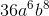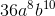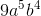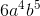Correct answer:Explanation:

Simplify:Step 1: Simplify the fraction. When dividing exponents subtract the exponents on the bottom from the exponents on the top.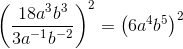Step 2: Distribute the exponent. When raising an exponent to a power, multiply them together.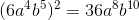### Example Question #1 : Exponential Operations

Simplify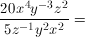Possible Answers: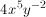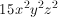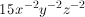None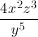Correct answer:Explanation:

Divide the coefficients and subtract the exponents.

### Example Question #1 : How To Divide Exponents

Which of the following is equal to the expression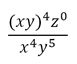, where

xyz ≠ 0?

Possible Answers:

z

xy

1/y

z/(xy)

xyz

Correct answer:

1/y

Explanation:

(xy)4 can be rewritten as x4y4 and z0 = 1 because a number to the zero power equals 1.  After simplifying, you get 1/y.

### Example Question #1 : How To Divide Exponents

If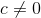, then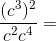Possible Answers: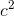Cannot be determined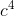Correct answer:Explanation:

Start by simplifying the numerator and denominator separately. In the numerator, (c3)2 is equal to c6. In the denominator, c2 * c4 equals c6 as well. Dividing the numerator by the denominator, c6/c6, gives an answer of 1, because the numerator and the denominator are the equivalent.

### Example Question #1 : How To Divide Exponents

If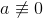, which of the following is equal to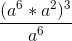?

Possible Answers:

The answer cannot be determined from the above information

a4

a6

a

a18

Correct answer:

a18

Explanation:

The numerator is simplified to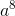(by adding the exponents), then cube the result. a24/a6 can then be simplified to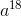.

← Previous 1

### All ACT Math Resources